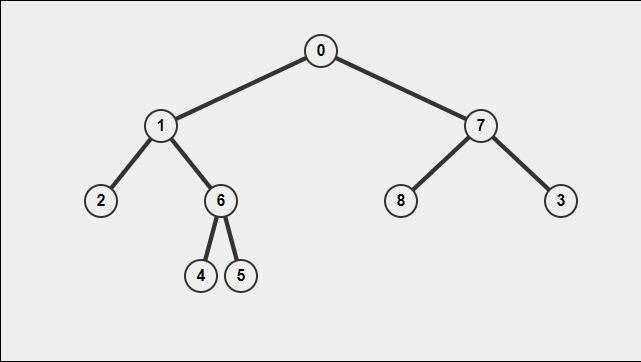Traversing binary trees is a fundamental skill required for coding interviews. Today I will teach you the main ways of traversing a binary tree. Each traversal style is associated with a specific pattern. Below is a quick reference. (All code examples are worked in python)

### Binary Tree Traversal Cheat Sheet

Traversal -> Pattern
In-order   left/center/right
pre-order   center/left/right
post-order   left/right/center
level-order   left to right

### Example#### Pre-Order Traversal

A preorder traversal follows the center-left-right pattern. For the tree above, the output would be [0,1,2,6,4,5,7,8,3]

##### Solution
def preOrderHelper(tree, array = []):
if tree != None:
array.append(tree.value)
preOrderHelper(tree.left,array)
preOrderHelper(tree.right,array)
return array


#### In-Order Traversal

An inorder traversal follows the left-center-right pattern. The algorithm will traverse as far left as possible and then add each center node with the right node being added last. An in-order traversal of the graph above would output [2,1,4,6,5,0,7,8,3]

##### Solution
def inOrder(tree, array = []):
if tree != None:
inOrderHelper(tree.left,array)
array.append(tree.value)
inOrderHelper(tree.right,array)
return array


#### Post-Order Traversal

A postorder traversal follows the left-right-center pattern. For the tree above the output would be [2,4,5,6,1,8,3,7,0].

##### Solution
def postOrder(tree, array = []):
if tree != None:
postOrderHelper(tree.left,array)
postOrderHelper(tree.right,array)
array.append(tree.value)


#### Level Order Traversal

A level order traversal will return a two dimensional list of every level in the tree. For the example above, the result would be:

[, [1,7], [2,6,8,3], [4,5]]

The code below uses a helper function. The helper function keeps track of which level of the tree it is located in.

##### Solution
def levelOrder(root: TreeNode) -> List[List[int]]:
if root == None:
return [[]]
acc = [[]]
helper(root,acc,0)
return acc

def helper(root,acc,ind):
if root == None:
return acc
else:
if len(acc) < ind + 1:
acc.append([])
acc[ind].append(root.val)
helper(root.left,acc,ind + 1)
helper(root.right,acc,ind + 1)
return acc